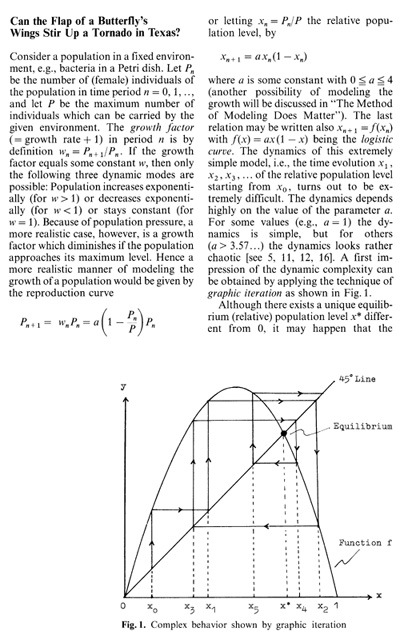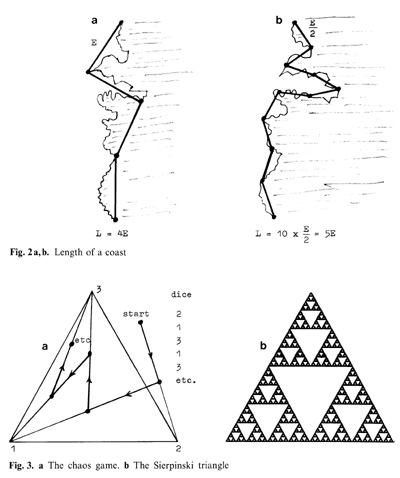On Some Concepts in Modeling Nonlinear Phenomena U. Krause     Hämatol. Bluttransf. Vol35
 FB Mathematik und Informatik, Universität Bremen, 2800 Bremen, FRG. Introduction Applying mathematics to problems in biology and medicine has a long history dating, at least, to the work of Fibonacci in the thirteenth century. In the twentieth century mathematical ideas have had a profound impact on population biology, genetics, epidemiology, and neurophysiology. However, in many ways, mathematical biology is still in its infancy. In such fields as molecular biology, cell biology, immunology, and certain branches of physiology, mathematics is just beginning to be recognized as a useful tool . Many exciting applications of mathematics to the life sciences are today concerned with nonlinear phenomena. Very often the relationship between variables is not linear by its very nature but it has to be treated linearly or by linear approximation because of the lack of mathematical tools handling the nonlinearity directly. Consider, for example, the, in the life sciences ubiquitous, phenomenon of growing ( or shrinking) populations (human beings, animals, plants, bacteria, cells, etc.). for carrying out the necessary mathematical analysis, a common idealization then is to assume a constant rate of growth. This is in contrast to the real dependence of the growth rate on the level of population, due to population pressure like crowding, abounded environment, food restrictions, etc. (see later sections for a more detailed discussion). During the last two decades there has been great success in developing new mathematical concepts and tools to cope with nonlinear phenomena (see the references given at the end of this paper; for applications in the life sciences, see especially [2, 4, 9, 11, 14, 17]). In particular, techniques were developed dealing with the by now famous concepts of "chaos", "fractals", and "cellular automaton" which have already entered many disciplines. However, these new developments have also shown that it may be extremely difficult to treat even the simplest kinds of nonlinearity analytically in a rigorous manner. Hence, another idea may be useful, which also arose in recent years and by which nonlinearities can be treated analytically if they come with certain properties of positivity, like positive and increasing levels of population. In contrast to what is possible for discrete nonlinear dynamic systems in general, the concept of positive dynamic systems also allows nonlinearities to be treated analytically in higher dimensions, i.e., for many variables. The aim of this contribution is only to offer a first impression for those Who are not yet acquainted with the concepts mentioned, but Who want to know roughly what they are about. I do not proceed in a mathematical manner by giving definitions and theorems, but discuss simple examples and illustrate the underlying ideas. The interested reader Who wants to know more is referred to the references given at the end of the paper.successive levels x1, x2, X3,... do not approach this equilibrium, but show a very irregular behavior with respect to it. Beside the parameter a the dynamic behavior depends very sensitively on the initial (relative) population Xo. This is a characteristic feature (but not the only one) of chaotic dynamic or (deterministic) chaos. More precisely, one speaks of sensitive dependence on initial condition,\' with respect to a dynamic system if a small change in the initial condition Xo may cause large differences in the course of time. This is the issue addressed by the famous quote of the meteorologist Edward Lorenz given in this sections title. The bad implications for predicting the behavior of a chaotic dynamic system are at hand. What is surprising is that such a simple and completely determined mechanism as the logistic curve may create a behavior which looks very much random. A long time ago, the logistic curve for a = 4 was used to generate random numbers . The Fractal Point Of View Nature presents itself rather differently from what mathematicians usually depict in geometry. Leaves, clouds, coasts, lungs, mountains, radiolaria, etc. are forms too complicated and too fantastic for describing them in terms of Euclidean geometry [3, 10]. Nevertheless, coping with these natural objects the scientist has to find some way to measure those objects. The difficulties of measuring natural forms in common terms have been pointed out by Benoit Mandelbrot (1967) in an article entitled "How long is the coast of Britain?" [cf. 10]. For measuring the true length one has to make the unit of measurement E smaller and smaller and then to take the limit case of all the lengths obtained. This does work even for curved objects when they are smooth enough but it does not work for "fractional" objects or fractals as are they named by Mandelbrot (for fractals see [1,10,12,13,15,17]). Measuring the fractional coast of Britain by making the unit of measurement smaller does increase the length obtained to infinity, as is indicated in Fig. 2. In the article mentioned, Mandelbrot refers to the British scientist Lewis fry Richardson who had already, in 1961, analyzed measurements made empirically for various coasts. Thereby he detected a magnitude not for the length of the coast but for the "roughness" of the coast, which was then called fractal dimension by Mandelbrot. More precisely, the fractal dimension measures (in the above example) how much the logarithm of the length L increases compared to an increase in the logarithm of the inverse unit of measurement 1/h.. To put it in a formula, the dependence of L on J:-' is given by the power law: L=cE 1-d where d is the fractal dimension and c is some constant. The fractal dimensions calculated for various coasts are strictly greater than 1 (which is the dimension of a straight line) and strictly smaller than 2 (which is the dimension of a plane). Today, the concepts of a fractal and of a fractal dimension are used to measure surfaces like those of lungs, blood vessel systems, or of materials in chemical reactions. There are several possibilities in giving a precise definition of a fractal. A fractal may be defined by the fact that the fractal dimension does not coincide with the dimension in the usual sense or it may also be defined by its self-similarity, (In fact, several types of fractals have to be distinguished.) Another possibility which, moreover, is very practical in constructing fractals using the computer is the chaos game invented by Barnsley [1 ] which uses a random process as in Fig. 3 a, b. Draw a (equilateral) triangle in the plane with vertices 1, 2, 3 as shown in Fig. 3 a and mark an arbitrary starting point in the plane. Throw a dice which is assumed to show the numbers 1, 2, 3 only because any two opposite faces of the dice are labeled with the same number.If the dice shows number n, then draw a line connecting the starting point with vertex 11 and obtain a new point by marking the middle point of it. Throw the dice again. If the dice shows number m, connect the new point obtained with vertex m by a line and mark on it the middle point as the new point. This process can be continued. What will be the figure formed by all the marked (middle) points? As it turns out, all these points arrange after a while on a classical fractal known for a long time as the Sierpinski triangle, shown in Fig. 3 b. The process described may also be considered as a dynamic system with the Sierpinski triangle as at tractor, that is, the motion of the system is finally attracted to the Sicrpinski triangle. Fractals appear as at tractors of nonlinear dynamic systems. (For the connection between fractals and dynamic systems as well as for an astonishing gallery oftive of f(x) = x/(c+x) remains positive throughout. (Related to this is the fact that overshooting Pn > P is admitted in this model but not in the first model given in this chapter.) The discussion shows that the method of modeling matters. The same qualitative description may be modeled differently in quantitative terms and then result in extremely different conclusions concerning the dynamic bchavior, like chaotic behavior versus stable behavior. Now, the example in Fig.4 is only a very simple one in the realm of positive discrete dynamic systems. The point in considering positive systems is that there are methods available which work also in higher dimensions, i.e., if many variables are involved [cf. 6, 8, 18]. Also, there are biological processes which arc in a natural way positive systems [for examples, see 6, 18]. For positive systems, too, the attract or may be a set, not just a single point, although this set will be not very complicated, in particular not a chaotic or strange at tractor, because of the properties of positivity . References 1. Barnsley M F (1988) Fractals everywhere. Acadcmic, Boston 2. Casti J L ( 1989) Alternate realities. Math ematical models of nature and man. Wiley, New York 3. D' Arcy Thompson W (1917) On growth and form. Cambridge University Press, Cambridge 4. Dembo M, Goldst(;in B, Perelson AS «(;ds) (1988) Nonlinearity in biology and medi cine. Math Biosci [Special issue] 90 5. Devaney RL (1986) An introduction to chaotic dynamical systems. Benjamin! Cummings, Menlo Park 6. Fujimoto T, Krause U (1988) Asymptotic properties for inhomogeneous iterations of nonlinear operators. SlAM J Math Anal 19:841-853 7. Hirsch M (1985) At tractors for discretetime monotone dynamical systems in strongly ordered spaces. In: Lecture Notes in Mathematics 1167. Springer, Berlin Heidelberg New York, pp141-153 8. Krause U (1986) A nonlinear extension of the Birkhoff-Jentzsch theor(;m. J Math Anal AppII14.552-568 9. Langton CG (ed) (1989) Artificial lifc. proce(;dings of an interdisciplinary workshop on the synthcsis and simulation of living systems. Addison-Wesley, New York (Santa Fe Institute studies in the sciences of complexity, vol6) 10. Mandelbrot B (1982) The fractal geometry of nature. Freeman, New York 11. May RM (1976) Simple mathematical models with very complicated dynamics. Nature 261 459-467 12. Peitgen HO, Richter PH (1986) The beauty of fractals. Images of complex dynamical systems. Springer, Berlin Heidelberg New York 13. Peitgen HO, Saup(; D (eds) (1988) The science of fractal images. Springer, Berlin Heidelberg New York 14. Perelson AS (ed) (1988) Theoretical immunology. Addison-Weslcy, New York (Santa Fe Institute studies in the sciences of complexity, vols 2, 3) 15. Ruelle D (1989) Chaotic evolution and strange at tractors. Cambridge University Press, Cambridge 16. Sandefur JT (1990) Discrete dynamical systems. Clarendon, Oxford 17. Schwietering J, Plath P (1990) Wachsende Muster. Preprint, Bremen 18. Smith HL (1986) Cooperative systcms of differential equations with concave nonlinearitics. Nonlinear Anal] 0: ]037 -]052 19. Ulam S, von Neumann J (1947) On com binations of stochastic and deterministic processes. Bull Am Math Soc 53.]120